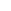# py内的文件代码为例（附案例）

05-17 159阅读

1、模块的四种方式

2、为什么要用模块？二、如何用模块

```# spam.py
print('from the spam.py')
money = 1000
print('spam模块：', money)
print('spam模块')
def change():
global money
money = 0```

1、模块名

`import module1[, module2[,... moduleN]`

```# run.py
import spam # from the spam.py
import spam
money = 111111
spam.change()
print(spam.money) # 0
print(money) # 111111```

```# run.py
import spam as sm
money = 111111
sm.money
sm.change()
print(money) # 1000```

```import spam, time, os
# 推荐使用下述方式
import spam
import time
import os```

2、from模块名具体的变量

`from modname import name1[, name2[, ... nameN]]`

from......导入的组件，访问不应该加前缀。

from......首次导入模块出现了3件事：

```# run.py
from spam import money
money = 10
print(money) # 10```

rom&;*语句：导入文件内所有的功能：

```# spam.py
# run.py
from spam import * # 导入spam.py内的所有功能，但会受限制于__all__
money = 111111
change()
print(money) # 111111```

3、循环导入

```# m1.py
print('from m1.py')
from m2 import x
y = 'm1'
# m2.py
print('from m2.py')
from m1 import y
x = 'm2'```

```# m1.py
print('from m1.py')
def func1():
from m2 import x
print(x)
y = 'm1'
# m2.py
print('from m2.py')
def func1():
from m1 import y
print(y)
x = 'm2'```

```5、# m1.py
print('from m1.py')
y = 'm1'
from m2 import x
# m2.py
print('from m2.py')
x = 'm2'
from m1 import y```

4、dir()函数

```dir(sys)
'__package__', '__stderr__', '__stdin__', '__stdout__',
'_clear_type_cache', '_current_frames', '_debugmallocstats', '_getframe',
'_home', '_mercurial', '_xoptions', 'abiflags', 'api_version', 'argv',
'base_exec_prefix', 'base_prefix', 'builtin_module_names', 'byteorder',
'dont_write_bytecode', 'exc_info', 'excepthook', 'exec_prefix',
'executable', 'exit', 'flags', 'float_info', 'float_repr_style',
'getcheckinterval', 'getdefaultencoding', 'getdlopenflags',
'getfilesystemencoding', 'getobjects', 'getprofile', 'getrecursionlimit',
'getrefcount', 'getsizeof', 'getswitchinterval', 'gettotalrefcount',
'gettrace', 'hash_info', 'hexversion', 'implementation', 'int_info',
'intern', 'maxsize', 'maxunicode', 'meta_path', 'modules', 'path',
'path_hooks', 'path_importer_cache', 'platform', 'prefix', 'ps1',
'setcheckinterval', 'setdlopenflags', 'setprofile', 'setrecursionlimit',
'setswitchinterval', 'settrace', 'stderr', 'stdin', 'stdout',

```a = [1, 2, 3, 4, 5]
import fibo
fib = fibo.fib
print(dir()) # 得到一个当前模块中定义的属性列表
# ['__builtins__', '__name__', 'a', 'fib', 'fibo', 'sys']
b = 5 # 建立一个新的变量 'a'
print(dir())
# ['__annotations__', '__builtins__', '__cached__', '__doc__', '__file__', '__loader__', '__name__', '__package__', '__spec__', 'a', 'b']
del b # 删除变量名a
print(dir())
# ['__annotations__', '__builtins__', '__cached__', '__doc__', '__file__', '__loader__', '__name__', '__package__', '__spec__', 'a']```

```# test.py
import m1 # 从m1.py文件中导入的,然后会生成m1模块的名称空间
import time
# 删除m1.py文件,m1模块的名称空间仍然存在
time.sleep(10)
import m1 # 不报错,一定不是从文件中获取了m1模块,而是从内存中获取的```

2、内置的模块

```# time.py
print('from time.py')
# run.py
import time
print(time) #```

3、环境变量sys.path中找（指出：sys.path的第一个值是当前执行文件的所在的文件夹）

```import sys
for n in sys.path:
print(n)
# C:\PycharmProjects\untitled\venv\Scripts\python.exe C:/PycharmProjects/untitled/hello.py
# C:\PycharmProjects\untitled
# C:\PycharmProjects\untitled
# C:\Python\Python38\python38.zip
# C:\Python\Python38\DLLs
# C:\Python\Python38\lib
# C:\Python\Python38
# C:\PycharmProjects\untitled\venv
# C:\PycharmProjects\untitled\venv\lib\site-packages```

```# run.py
import sys
sys.path.append(r'C:\PycharmProjects\untitled\day16')
print(sys.path)
import mmm
mmm.f1()```

2、搜索模式以执行文件为准

```from aa import spam
print(spam.money)```

1、当run.py运行的之后，aaa.py被当作引用模块，它的=='aaa'（模块名），会执行aaa.py中的f1()。

```# aaa.py
x = 1
def f1():
print('from f1')
f1()
# run.py
import aaa```

2、aaa.py被只是可执行文件时，加上==''，单独运行aaa.py才会执行aaa.py中的f1()。run.py运行时可以避免执行f1()。

```# aaa.py
x = 1
def f1():
print('from f1')
if __name__ == '__main__':
f1()```

1、两种方法导入：

2、导入包内的模块

```import aaa.bbb.m3
print(aaa.bbb.m3.func3())```

3、from方式：导入模块内详细的模块

```from aaa.bbb import m3
print(m3.func3())```

```from aaa.bbb.m3 import func3
print(func3())```

4、绝对导入和相对导入绝对导入：

```# aaa/.py
from aaa.m1 import func1
from aaa.m2 import func2```

```from .m1 import func1
from .m2 import func2```

5、from...*

`__all__ = ["echo", "surround", "reverse"]`

```ATM/
|-- core/
| |-- src.py # 业务核心逻辑代码
|
|-- api/
| |-- api.py # 接口文件
|
|-- db/
| |-- db_handle.py # 操作数据文件
| |-- db.txt # 存储数据文件
|
|-- lib/
| |-- common.py # 共享功能
|
|-- conf/
| |-- settings.py # 配置相关
|
|-- bin/
| |-- run.py # 程序的启动文件，一般放在项目的根目录下，因为在运行时会默认将运行文件所在的文件夹作为sys.path的第一个路径，这样就省去了处理环境变量的步骤
|
|-- log/
| |-- log.log # 日志文件
|

.py

```# settings.py
import os
BASE_DIR = os.path.dirname(os.path.dirname(os.path.abspath(__file__)))
DB_PATH = os.path.join(BASE_DIR, 'db', 'db.txt')
LOG_PATH = os.path.join(BASE_DIR, 'log', 'user.log')
# print(DB_PATH)
# print(LOG_PATH)```

.py

```# common.py
import time
from conf import settings
def logger(msg):
current_time = time.strftime('%Y-%m-%d %X')
with open(settings.LOG_PATH, mode='a', encoding='utf-8') as f:
f.write('%s %s' % (current_time, msg))```

src.py

```# src.py
from conf import settings
from lib import common
print('登陆')
def register():
print('注册')
with open(settings.DB_PATH, mode='a', encoding='utf-8') as f:
f.write('%s:%s\n' % (name, pwd))
# 记录日志。。。。。。
common.logger('%s注册成功' % name)
print('注册成功')
def shopping():
print('购物')
def pay():
print('支付')
def transfer():
print('转账')
func_dic = {
'2': register,
'3': shopping,
'4': pay,
'5': transfer,
}
def run():
while True:
print("""
1 登陆
2 注册
3 购物
4 支付
5 转账
6 退出
""")
choice = input('>>>: ').strip()
if choice == '6': break
if choice not in func_dic:
print('输入错误命令，傻叉')
continue
func_dic[choice]()```

run.py

```# run.py
import sys
import os
BASE_DIR = os.path.dirname(os.path.dirname(os.path.abspath(__file__)))
sys.path.append(BASE_DIR)
from core import src
if __name__ == '__main__':
src.run()```

• 1.注意：本站资源多为网络收集，如涉及版权问题请及时与站长联系，我们会在第一时间内删除资源。
• 2.您购买的只是资源，不提供解答疑问和安装服务。免费源码里的接口不保证一直可以用
• 3.本站用户发帖仅代表本站用户个人观点，并不代表本站赞同其观点和对其真实性负责。
• 4.本站资源大多存储在云盘，如发现链接失效，请及时与站长联系，我们会第一时间更新。
• 5.转载本网站任何内容，请按照转载方式正确书写本站原文地址
• 6.如果发现侵权可以联系站长删除站长QQ3326096692或者邮箱通知3326096692@qq.com
• #### 发表评论

快捷回复： 表情：
评论列表 （暂无评论，159人围观）

## 还没有评论，来说两句吧...

### 目录[+]请先 登录 再评论，若不是会员请先 注册